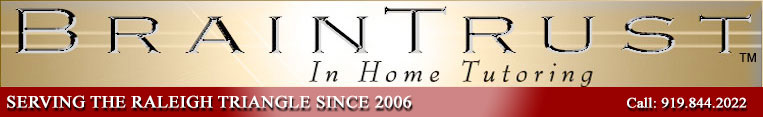## Algebra Tutoring

Client Quote

"I've heard that really good teachers like to get feedback... so here goes:
• You tutored _____ one time
• You made her laugh
• You helped her learn
• You increased her confidence
Less than 12 hours after your encounter _____ took a very important end of year exam (last one before finals). She got an 89. No way that happens without you!! Sincere thanks."
- Cary High School Math Student

Overview

Pre-Algebra, Algebra I, and Algebra II generally involve the process of solving for unknown quantities and understanding how to graph equations. BrainTrust tutors work with their students one-on-one to ensure the student understands what information each problem seeks to find.

" Our first session went very well, thank you! "
Middle School Support
Pre-Algebra

For some students, this is a big leap from basic arithmetic. With practice, students learn to isolate expressions and solve for the unknown.

In helping students solve equations, students have to learn, in these beginning stages, how to move forward to solutions even when they feel lacking in information. Pre-Algebra students need to build confidence working with variables, brackets and two-step processes. Absolute inequalities and order of operations must be fully understood before math students may advance to the higher levels of algebra.

Algebra I

Once students gain confidence solving for the unknown, "x." in problems, they will be asked to find the answer for an additional variable, "y." Graphs become more advanced in Algebra I. Students build on the material they learned in Pre-Algebra and practice mastering the quadratic formula. Especially important is the ability to work with integers. -- using commutative, associative and distributive properties to simplify equations.

As with all BrainTrust tutoring, sessions are initially geared to the subject material being currently presented in the student's core curriculum, with a focus upon homework problems, quiz material, study guides and comprehensive exam and test-taking strategies.Algebra II

Algebra II students will already have grasped easier equations. The more challenging skills of identification, application and generation (the highest level) will prepare students for more advanced studies in geometry and calculus, while ensuring higher scores on both end of grade exams, standardized math tests and SAT mathematical skills.

This involves simplifying and performing operations with radical expressions and expressions involving rational exponents, logarithms and calculators for higher order computations. Students define complex numbers, perform basic operations, write equations concerning circles and parabolas, and, eventually, graph the data.

BrainTrust tutors work with their students to graphically, algebraically and verbally describe real-world phenomena such as functions. Questions concerning identification of independent and dependent variables are addressed. Finally, students of Algebra II should be able to write and interpret an equation of a curve (e.g., linear, exponential, quadratic).

For more specific information, call (919) 844-2022 to set up an Algebra Program for your student.